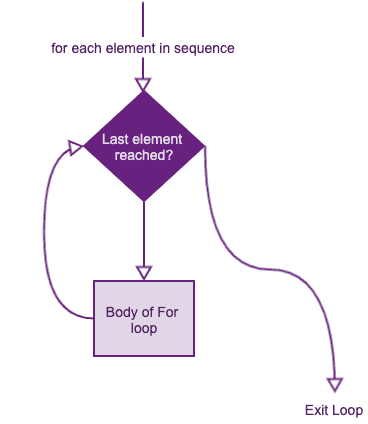# R For Loop (6 Examples)

A for loop in R is a way to repeat a code block for each item in a collection of objects, such as a vector, a list, or a dataframe. For example, for loop is used to iterate over a vector, executing each element within a series.

Syntax of for loop

``````for (item in sequence)
{
statement
}``````

The code in the body is executed until the end of the series is reached. The execution stops after the last item of the series is processed.

The sequence is a vector, and the item takes on each of its values during the loop. In each iteration, the statement is evaluated.

### Flow chart of for loopIn the flow chart, rectangular boxes mean “do something which does not imply decisions”.

The diamonds, on the other hand, are called “decision symbols” and therefore interpreted into questions that only have two possible logical answers, precisely, TRUE(T) or FALSE(F).

In general, the for loop comprises the rectangular box ‘init’, the diamond or rhombus decision, and the rectangular box is executed a known number of times.

### Example

For loop in R is incredibly flexible because they are not limited to integers or even numbers in the input. We can pass character vectors, logical vectors, lists, or expressions.

``````rv <- letters[1:4]
for (i in rv) {
print(i)
}``````

Output

`````` "a"
 "b"
 "c"
 "d"``````

Let’s see another example.

``````# Create shows vector
shows <- c("Mandalorian", "Lacasa De Papel", "Expanse", "Friends")

# Create the for statement
for (show in shows) {
print(show)
}``````

In the above example, the loop iterates four times as the vector shows vector has four elements.

In each iteration, the show takes on the value of the corresponding item of shows.

### Example 2: For loop over a list

Looping over a list is just as easy and convenient as looping over a vector. Let’s see the following code example.

``````data <- list(Shares = c("Reliance", "TCS", "HDFC Bank", "HUL"))

for (i in data) {
print(data)
}``````

Output

``````\$Shares
 "Reliance" "TCS" "HDFC Bank" "HUL"``````

### Example 3: For loop in the data frame

To loop over a data frame, you need to create a data frame and then use for loop to iterate it.

``````name <- c("Donald", "Joe", "Hillary")
age <- c(74, 78, 65)
city <- c("New york", "Denver", "Dallas")

df <- data.frame(name, age, city)

for (i in names(df)) {
print(df[i])
}``````

Output

`````` name
1 Donald
2 Joe
3 Hillary
age
1 74
2 78
3 65
city
1 New york
2 Denver
3 Dallas``````

You can see that loop prints one by one column of the data frame using for loop.

### Example 4: For loop with an increment in R

You can use the seq() function to increment the value in each iteration of the for loop.

``````for (i in seq(0, 20, 5)) {
print(i)
}``````

Output

`````` 0
 5
 10
 15
 20``````

### Example 5: Next statement in for loop

You can use the next statement for loop when you want to skip the execution based on a specific condition without stopping the entire loop’s execution.

``````for (i in seq(0, 20, 5)) {
if (i == 15) {
next
}
print(i)
}``````

#### Output

`````` 0
 5
 10
 20``````

### Example 6: Nested For Loops in R

Nested for loop means the for loop inside another for loop. For example, suppose you want to manipulate a bi-dimensional array by setting its elements to specific values. Then you should consider nesting the for loop.

``````mtrx <- matrix(nrow = 30, ncol = 30)

# For each row and for each column, assign values
# based on position: product of two indexes
for (i in 1:dim(mtrx)) {
for (j in 1:dim(mtrx)) {
mtrx[i, j] = i * j
}
}

# Display the upper left 5x5 chunk
mtrx[1:5, 1:5]``````

Output

``````    [,1] [,2] [,3] [,4] [,5]
[1,]  1    2    3    4    5
[2,]  2    4    6    8   10
[3,]  3    6    9    12  15
[4,]  4    8    12   16  20
[5,]  5    10   15   20  25``````

You have two nested for loops in the code chunk above and thus two sets of curly braces, each with its block and governed by its index. That is, i run over the lines, and j runs over the columns.